# More Advanced Tutorial for Rotations with Translations

#### Grandma Bates

##### Only mostly banned....
VVO Supporter 🍦🎈👾❤
This is an introduction to the use of rotations and translations in an LSL script. The goal here is to show how rotations and movement of a prim can be used together to make a prim appear to pivot around a point. It is a limited example, but hopefully this demonstration will motivate how a prim can appear to go through complex movements using a few simple ideas.

It is assumed that you are familiar with vectors and vector operations in LSL. It is also assumed that you are familiar with the basics of rotations. This is a follow up tutorial to the basic introduction: Basic Introduction to Rotations in LSL

The focus of the previous tutorial is on rotations. The real power of rotations can be realized when combined with translations. The idea is that you rotate a prim/object and then move it to a new location. (The order matters for technical reasons.) The idea of combining a rotation and a translation is an important idea in mathematics and has its own name, an Affine transformation. (See Affine transformation - Wikipedia, the free encyclopedia for more details.)

A prim can be rotated and moved in one step in LSL. The command to use is the llSetLinkPrimitiveParamsFast command. (see LlSetLinkPrimitiveParamsFast - Second Life Wiki for more details.) The basic format for the command we use here is the following:
Java:
``````llSetLinkPrimitiveParamsFast(linkNumber,[PRIM_ROTATION,theRot,
PRIM_POSITION,thePos]);``````
The variable "linkNumber" is the link number of the prim to move. The variable "theRot" is the rotation to set on the prim. The variable "thePos" is the new location to move the prim to. If the prim is the root prim then it will use the sim coordinates, otherwise it is the offset relative to the root prim.

A full compliment of vector operations is available in LSL, but here we only use vector addition. Vector addition can be thought of geometrically by arranging the vectors head to tail. (See Figure 1.) To subtract two vectors you "flip" the vector being subtracted. (See Figure 2.)

Figure 1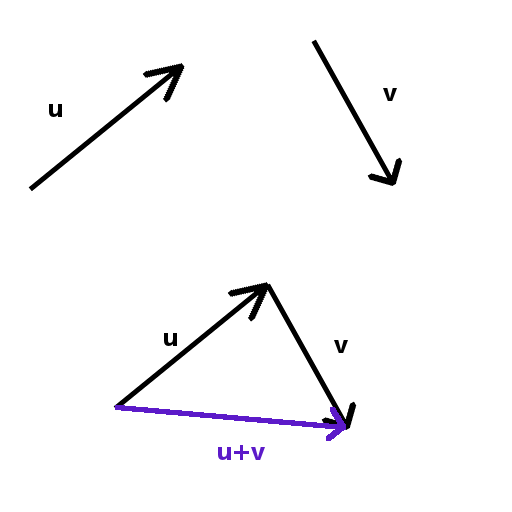Figure 2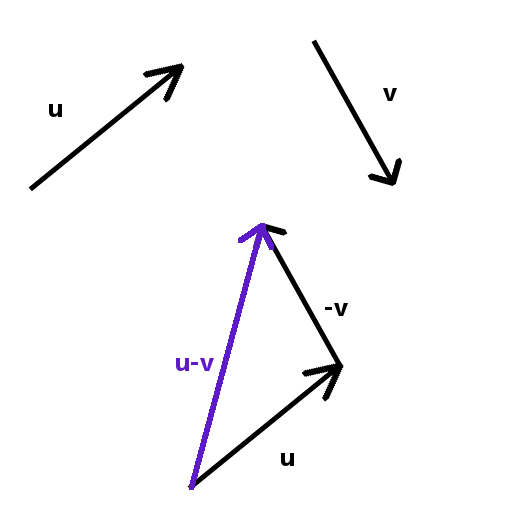If you multiply a vector by a rotation in LSL the result is the vector obtained by taking the vector and rotating it. (See Figure 3.) In the example the rotation, A, is a 45 degree rotation around the z-axis. The vector, x=<1,0,0>, is one unit in the x-direction. Imagine you are looking straight down from above in the z-direction. The vector times the rotation results in a vector that is pointing into the first quadrant.

Figure 3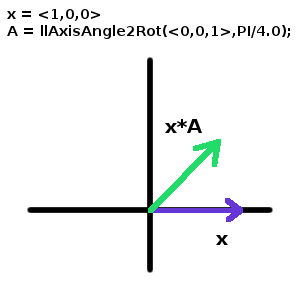We look at an example to illustrate how to combine rotations and translations. We will rotate a cylinder around its lower end. The subtlety is that the prim's position is defined to be its center. We need to define the rotation for the cylinder's new orientation and then calculate the new center associated with the new rotation. The first step is to find the center and rotation of the prim. The second step is to calculate the pivot point. Finally, the new position is found to rotate the cylinder 45 degrees around its local x-axis. (The idea is shown graphically in Figures 4 and 5.)

Figure 4 (Before the pivot.)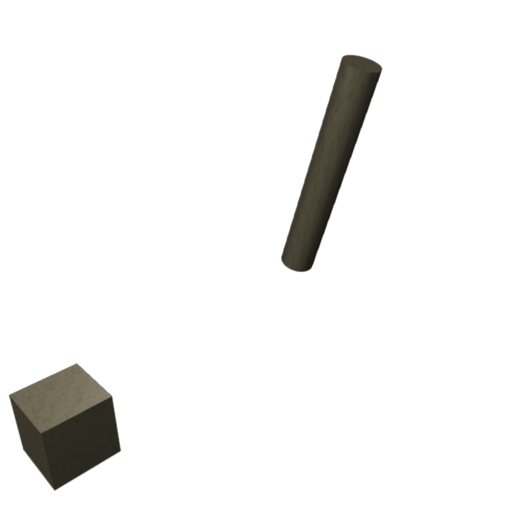Figure 5 (After the pivot.)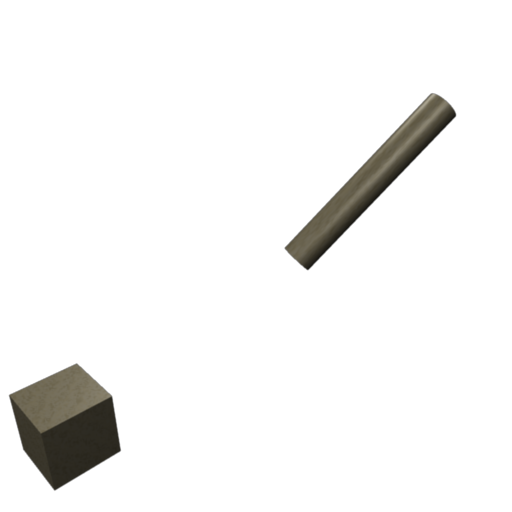To find the prim's position we need to consider the build. We assume that the cylinder is in a link set and is not the root prim. The name of the prim is "rotate me." The code to find the prim's orientation is given below:

Code:
``````rotation cylRot;
vector cylPos;
vector cylSize;

rotation homeRot = llGetRootRotation();   // The root object's sim rotation.
vector homePos = llGetRootPosition();     // The root object's sim position.
integer lupe = llGetNumberOfPrims()+1;

while(--lupe > 1)
{
{
cylRot = llList2Rot(params,1)/homeRot;              // The cylinder's local rotation.
cylPos = (llList2Vector(params,0)-homePos)/homeRot; // The cylinder's local position.
cylSize = llList2Vector(params,2);                  // The size of the cylinder.
lupe = -1;
}
}

{
return;
}``````
(I recognize that this code and the examples that follow are not optimal but are given to try to make the ideas clearer.)

We now have the center of the prim and its rotation. The next step is to calculate the pivot point (See Figures 6). To do this we need to find the vector that points along the cylinder's local z-axis and has a length 1/2 the height of the cylinder. The vector is found by multiplying a vector of the right length that points in the z-direction by the cylinder's rotation:

Code:
``vector cylHeight = <0.0,0.0,cylSize.z*0.5>*cylRot;``
The pivot point can be found by subtracting the length vector from the prim`s position:

Code:
``vector pivot = cylPos - cylHeight;``
Figure 6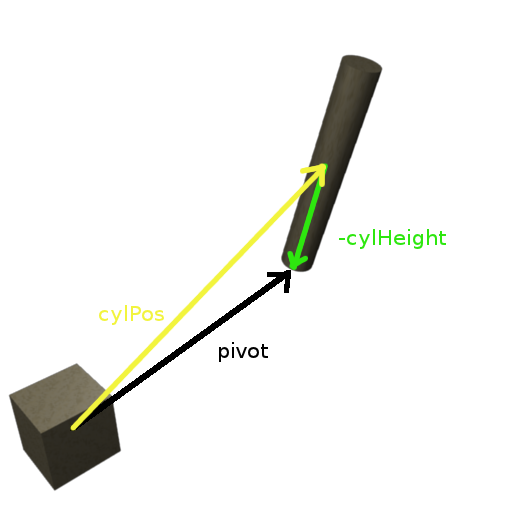Finally the rotation for the new orientation is found. We want to rotate the prim around its local x-axis (See Figure 7). That means we want to use a left multiplication. The new rotation and position is defined in the following code:

Code:
``````rotation newRot = llAxisAngle2Rot(<1.0,0.0,0.0>,PI/4.0);
PRIM_POSITION,pivot+<0.0,0.0,cylSize.z*0.5>*newRot*cylRot]);``````
Figure 7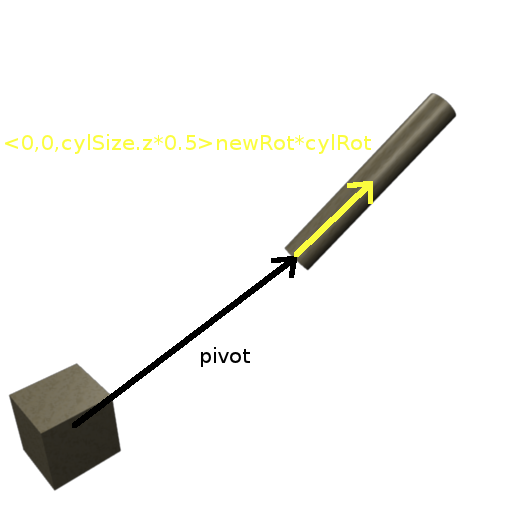We leave it as an exercise to rotate a box around one edge for a door script. For extra credit make it rotate slowly using the idea from the previous tutorial. Also, make sure it is around an edge and not the middle of a side face. (This is one of the author's pet peeves to see a door that does not rotate around an edge but around one end in the middle of a face. Ugh!)

• 3• 2